# Python: Linear Regression

Regression is still one of the most widely used predictive methods. If you are unfamiliar with Linear Regression, check out my: Linear Regression using Excel lesson. It will explain the more of the math behind what we are doing here. This lesson is focused more on how to code it in Python.

We will be working with the following data set: Linear Regression Example File 1

## Import the data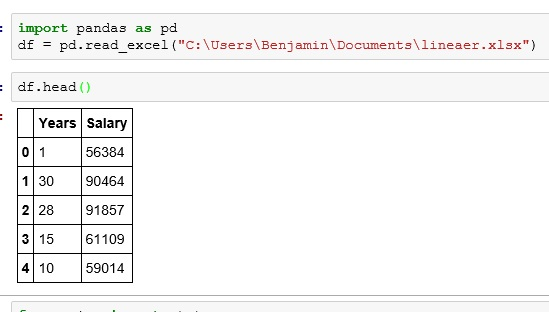What we have is a data set representing years worked at a company and salary.

## Let’s plot it

Before we go any further, let’s plot the data.

Looking at the plot, it looks like there is a possible correlation.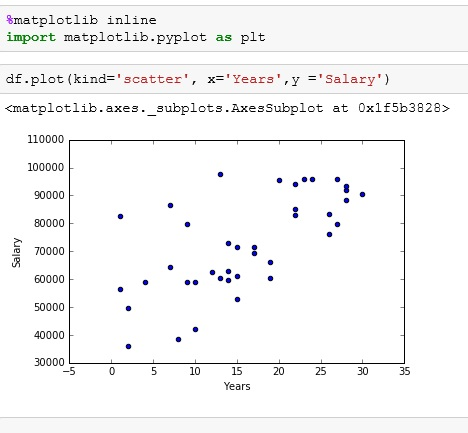## Linear Regression using scipy

scipy library contains some easy to use maths and science tools. In this case, we are importing stats from scipy

the method stats.linregress() produces the following outputs: slope, y-intercept, r-value, p-value, and standard error.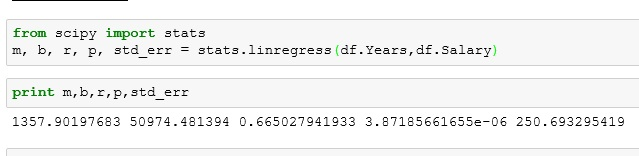I set slope to m and y-intercept to b: so we match the linear formula y = mx+b

Using the results of our regression, we  can create an easy function to predict a salary. In the example below, I want to predict the salary of a person who has been working there 10 years.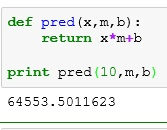Our p value is nice and low. This means our variables do have an effect on each other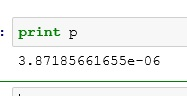Our standard error is 250, but this can be misleading based on the size of the values in your regression. A better measurement is r squared. We find that by squaring our r outputR squared runs from 0 (bad) to 1 (good). Our R squared is .44. So our regression is not that great. I prefer to keep a r squared value at least .6 or above.

## Plot the Regression Line

We can use our pred() function to find the y-coords needed to plot our regression line.

Passing pred() a x value of 0 I get our bottom value. I pass pred() a x value of 35 to get our top value.

I then redo my scatter plot just like above. Then I plot my line using plt.plot().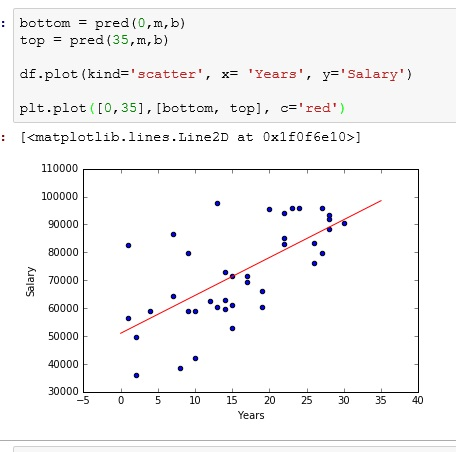If you enjoyed this lesson, click LIKE below, or even better, leave me a COMMENT.